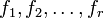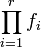# Schinzel's hypothesis H

## Statement

Supposeare all irreducible polynomials with integer coefficients and with positive leading coefficient, such that the productdoes not have any fixed divisors, i.e., it cannot be expressed as a proper multiple of an integer-valued polynomial. Schinzel's hypothesis H states that there are infinitely many natural numberssatisfying the condition thatare all simultaneously prime.

## Related facts and conjectures

### Weaker facts and conjectures

Fact or conjecture Status How it fits with Schinzel's hypothesis H
Dickson's conjecture Open We are dealing with linear polynomials.
Bunyakovsky conjecture open We are dealing with only one irreducible polynomial of degree two or higher
Dirichlet's theorem on primes in arithmetic progressions proved We are dealing with one irreducible polynomial of degree one
Twin prime conjecture open We are dealing with the irreducible polynomialsand. Also, the dependence is via Dickson's conjecture.
Green-Tao theorem proved The theorem states that the sequence of primes contains arithmetic progressions of arbitrary length. The Green-Tao theorem can be viewed as a corollary of Schinzel's hypothesis H if we view it as a collection of statements, one about the existence of arithmetic progressions of a specific length. Each such statement is substantially weaker than what we'd get from Schinzel's hypothesis H (which not only guarantees the existence of such arithmetic progressions, but also allows us to choose a common difference for the arithmetic progression that satisfies some divisibility conditions). The dependence is via Dickson's conjecture.